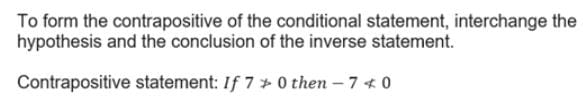Test: Implications And Validating Of Statements

# Test: Implications And Validating Of Statements - Commerce

Test Description

## 10 Questions MCQ Test Mathematics (Maths) Class 11 - Test: Implications And Validating Of Statements

Test: Implications And Validating Of Statements for Commerce 2023 is part of Mathematics (Maths) Class 11 preparation. The Test: Implications And Validating Of Statements questions and answers have been prepared according to the Commerce exam syllabus.The Test: Implications And Validating Of Statements MCQs are made for Commerce 2023 Exam. Find important definitions, questions, notes, meanings, examples, exercises, MCQs and online tests for Test: Implications And Validating Of Statements below.
Solutions of Test: Implications And Validating Of Statements questions in English are available as part of our Mathematics (Maths) Class 11 for Commerce & Test: Implications And Validating Of Statements solutions in Hindi for Mathematics (Maths) Class 11 course. Download more important topics, notes, lectures and mock test series for Commerce Exam by signing up for free. Attempt Test: Implications And Validating Of Statements | 10 questions in 10 minutes | Mock test for Commerce preparation | Free important questions MCQ to study Mathematics (Maths) Class 11 for Commerce Exam | Download free PDF with solutions
 1 Crore+ students have signed up on EduRev. Have you?
Test: Implications And Validating Of Statements - Question 1

### Two pairs of statement are: p: If a quadrilateral is a rectangle, then its opposite sides are equal. q: If opposite sides of a quadrilateral are equal, then the quadrilateral is a rectangle. The combined statement of these pairs using “If and only if” is:

Detailed Solution for Test: Implications And Validating Of Statements - Question 1

‘A quadrilateral is a rectangle if and only if its opposite sides are equal’ because statements p & q are satisfied in this combined statement.

Test: Implications And Validating Of Statements - Question 2

### Write the contra positive of the given statement If -7 < 0 then 7 > 0

Detailed Solution for Test: Implications And Validating Of Statements - Question 2Test: Implications And Validating Of Statements - Question 3

### The contra positive of a statement p ⇒ q, is the statement

Detailed Solution for Test: Implications And Validating Of Statements - Question 3

Contraceptive of a→b is ∼b→∼a
Then,Contraceptive of p→(∼q→∼r)
≡∼(∼q→∼r)→∼p
≡∼(q∧∼r)→∼p[a→b≡∼a∧b]
≡(∼q∨r)→∼p  [Demorgas law]

Test: Implications And Validating Of Statements - Question 4

Name the technique used in the first step of the soluntion of the problem below :
Verify that √5 is irrational
Solution ; Let us assume that √5 is rational

Test: Implications And Validating Of Statements - Question 5

If p and q are mathematical statements, then in order to show that the statement “p and q” is true, we need to show that:

*Multiple options can be correct
Test: Implications And Validating Of Statements - Question 6

In order to prove the statement “If p then q” we need to show that:

Detailed Solution for Test: Implications And Validating Of Statements - Question 6

Case 1: By assuming that p is true, prove that q must be true.(Direct method)
Case 2 :  By assuming that q is false, prove that p must be false.(Contrapositive Method)

Test: Implications And Validating Of Statements - Question 7

In order to prove the statement “p if and only if q” we need to show:

Detailed Solution for Test: Implications And Validating Of Statements - Question 7

In order to prove the statement “p if and only if q”, we need to show.

• If p is true, then q is true and
• If q is true, then p is true.
Test: Implications And Validating Of Statements - Question 8

The component statements are:
p: You are wet when it rains.
q: You are wet when you are in river.
The compound statement of these component statements using appropriate connective is:

Test: Implications And Validating Of Statements - Question 9

Which option is not same as “if p then q”?

Test: Implications And Validating Of Statements - Question 10

Name the technique used in the solution of the problems below :
Question: Show that the following statement is false: If n is an odd integer, then n is prime.
Solution: The given statement is in the form “if p then q” we have to show that this is false, If p then ~q.
n = 99 is odd integer which is not a prime number. Thus, we conclude that the given statement is false.

Detailed Solution for Test: Implications And Validating Of Statements - Question 10

Here n = 99 is a counter example of the given statement.
Therefore,
Option A

## Mathematics (Maths) Class 11

157 videos|210 docs|132 tests
Information about Test: Implications And Validating Of Statements Page
In this test you can find the Exam questions for Test: Implications And Validating Of Statements solved & explained in the simplest way possible. Besides giving Questions and answers for Test: Implications And Validating Of Statements, EduRev gives you an ample number of Online tests for practice

## Mathematics (Maths) Class 11

157 videos|210 docs|132 tests(Scan QR code)